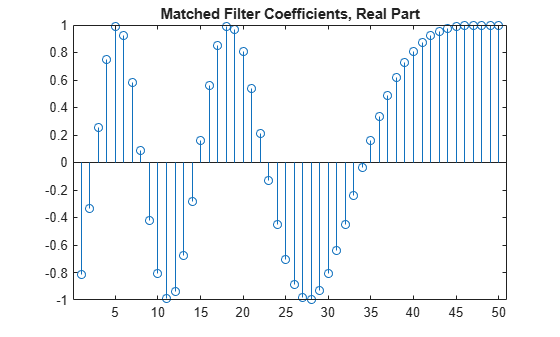getMatchedFilter

System object: phased.LinearFMWaveform
Package: phased

Matched filter coefficients for waveform

Syntax

Coeff = getMatchedFilter(H)
Coeff = getMatchedFilter(H,'FrequencyOffset',FOFFSET)

Description

Coeff = getMatchedFilter(H) returns the matched filter coefficients for the linear FM waveform object H. Coeff is a column vector.

Coeff = getMatchedFilter(H,'FrequencyOffset',FOFFSET) adds a frequency offset when matched filter coefficients are generated. FOFFSET must be a scalar. This option is available when you set the FrequencyOffsetSource property to 'Input port' for the input object, H.

Examples

expand all

Get the matched filter coefficients for a linear FM pulse.

waveform = phased.LinearFMWaveform('PulseWidth',5e-05,...
'SweepBandwidth',1e5,'OutputFormat','Pulses');
coeff = getMatchedFilter(waveform);
stem(real(coeff))
title('Matched Filter Coefficients, Real Part')